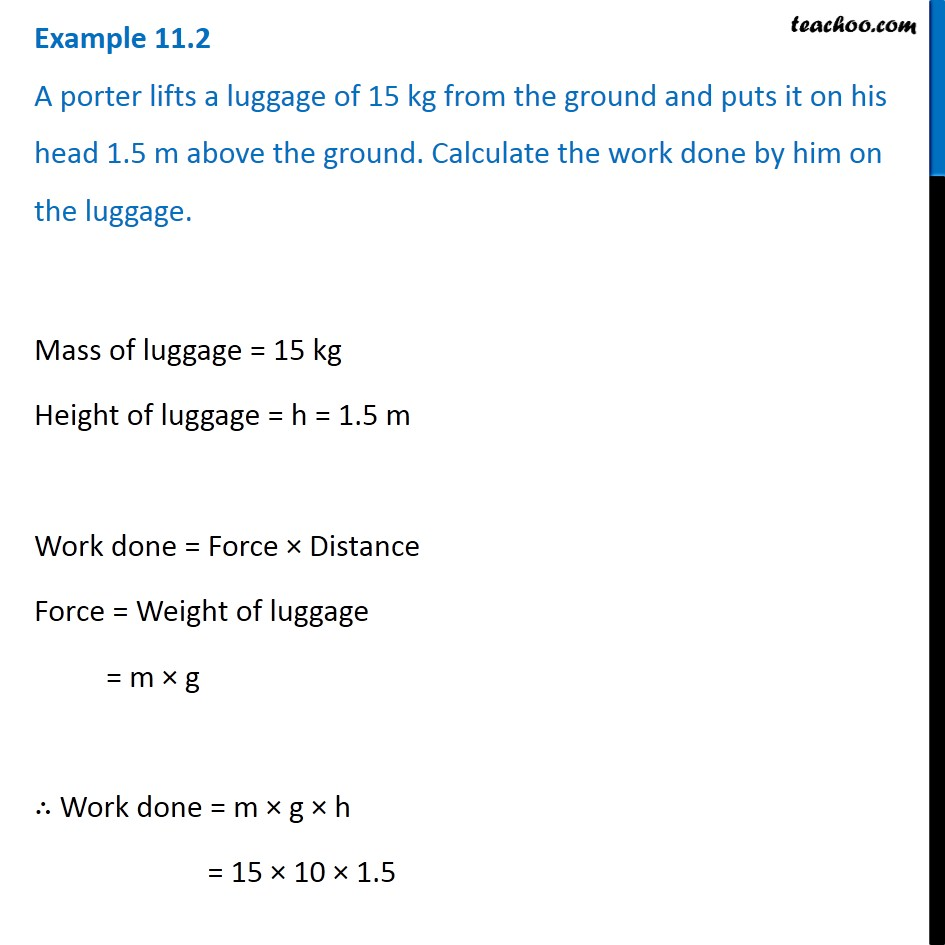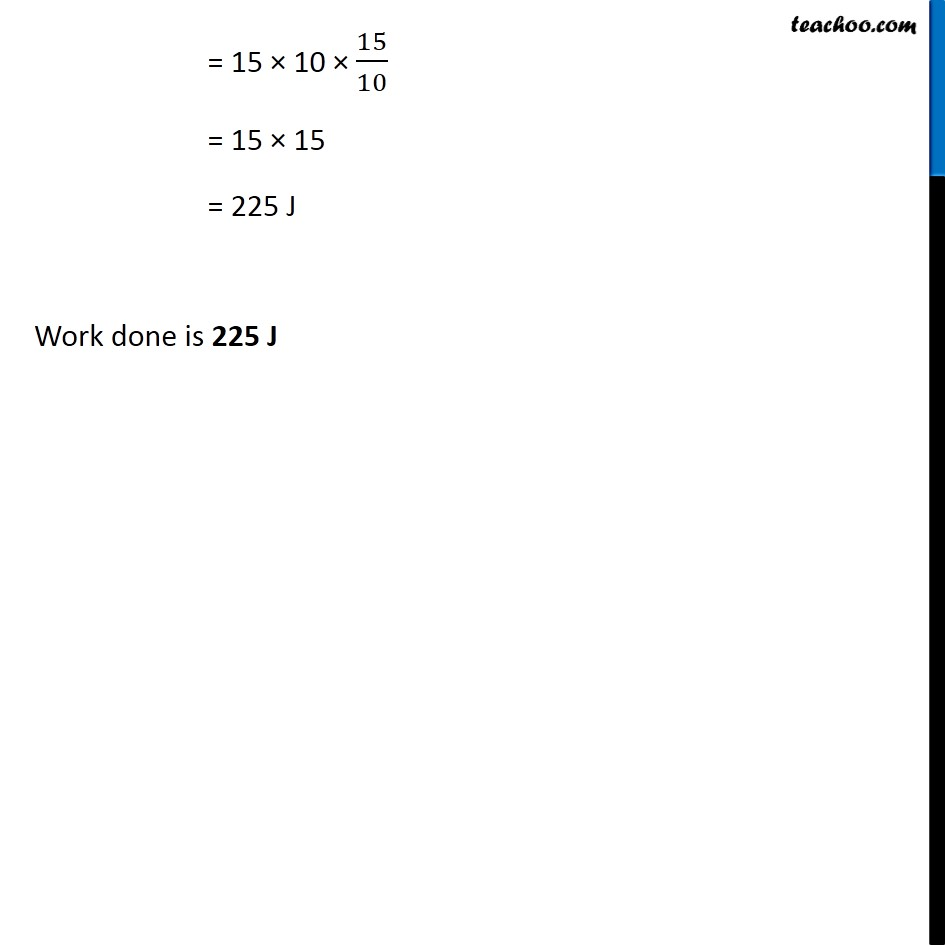Examples from NCERT Book

Class 9
Chapter 11 Class 9 - Work and EnergyLearn in your speed, with individual attention - Teachoo Maths 1-on-1 Class

### Transcript

Example 11.2 A porter lifts a luggage of 15 kg from the ground and puts it on his head 1.5 m above the ground. Calculate the work done by him on the luggage. Mass of luggage = 15 kg Height of luggage = h = 1.5 m Work done = Force × Distance Force = Weight of luggage = m × g ∴ Work done = m × g × h = 15 × 10 × 1.5 = 15 × 10 × 15/10 = 15 × 15 = 225 J Work done is 225 J